### D.32 In­te­gral con­ser­va­tion laws

This sec­tion de­rives the in­te­gral con­ser­va­tion laws given in ad­den­dum {A.14}.

The rules of en­gage­ment are as fol­lows:

• The Carte­sian axes are num­bered us­ing an in­dex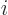, with1, 2, and 3 for,, and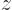re­spec­tively.
• Also,in­di­cates the co­or­di­nate in thedi­rec­tion,,, or.
• De­riv­a­tives with re­spect to a co­or­di­nateare in­di­cated by a sim­ple sub­script.
• Time de­riv­a­tives are in­di­cated by a sub­script t.
• A bare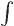in­te­gral sign is as­sumed to be an in­te­gra­tion over all space, or over the en­tire box for par­ti­cles in a box. The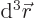is nor­mally omit­ted for brevity and to be un­der­stood.
• A su­per­script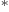in­di­cates a com­plex con­ju­gate.

First it will be shown that ac­cord­ing to the Schrö­din­ger equa­tion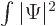is con­stant. The Schrö­din­ger equa­tion in free space is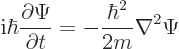Tak­ing the right hand term to the other side and writ­ing it in in­dex no­ta­tion gives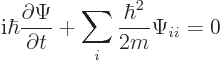Mul­ti­ply the left hand side by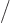and add the com­plex con­ju­gate of the same equa­tion to getTo show that the in­te­gralis con­stant, it must be shown that its time de­riv­a­tive is zero. Now the first two terms above are the time de­riv­a­tive of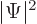. So in­te­grated over all space, they give the time de­riv­a­tive that must be shown to be zero. And the equa­tion above shows that it is in­deed zero pro­vided that the re­main­ing sum in it in­te­grates to zero over all space.

The con­stant is not im­por­tant in show­ing that this is true, so just ex­am­ine for anyThis equals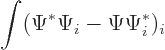as can be seen by dif­fer­en­ti­at­ing out the par­en­thet­i­cal ex­pres­sion with re­spect to. The above in­te­grand can be in­te­grated with re­spect to. It will then be zero for a pe­ri­odic box since the ex­pres­sion in paren­the­sis is the same at the up­per and lower lim­its of in­te­gra­tion. It will also be zero for an im­pen­e­tra­ble con­tainer, since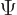will then be zero on the sur­face of the con­tainer. It will also be zero in an in­fi­nite re­gion pro­vided thatand its de­riv­a­tives van­ish at large dis­tances.

There is an­other way to see thatis con­stant. First re­call that any so­lu­tion of the Schrö­din­ger equa­tion takes the form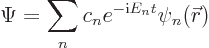Here theare the en­ergy eigen­func­tions. ThenNow be­cause of or­tho­nor­mal­ity of the eigen­func­tions, the in­te­gra­tion only pro­duces a nonzero re­sult when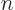, and then the prod­uct of the eigen­func­tions in­te­grates to 1. So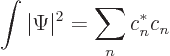That does not de­pend on time, and the nor­mal­iza­tion re­quire­ment makes it 1.

This also clar­i­fies what goes wrong with the Klein-Gor­don equa­tion. For the Klein-Gor­don equa­tion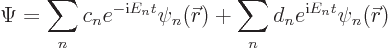The first sum are the par­ti­cle states and the sec­ond sum the an­tipar­ti­cle states. That gives: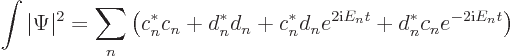The fi­nal two terms in the sum os­cil­late in time. So the in­te­gral is no longer con­stant.

The ex­cep­tion is if there are only par­ti­cle states (no) or only an­tipar­ti­cle states (no). In those two cases, the in­te­gral is con­stant. In gen­eral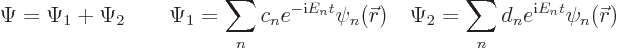where the in­te­grated square mag­ni­tudes of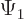andare con­stant.

Next it will be shown that the re­arranged Klein-Gor­don equa­tion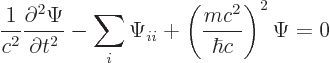pre­serves the sum of in­te­gralsTo do so it suf­fices to show that the sum of the time de­riv­a­tives of the three in­te­grals is zero. That can be done by mul­ti­ply­ing the Klein-Gor­don equa­tion by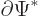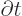, adding the com­plex con­ju­gate of the ob­tained equa­tion, and in­te­grat­ing over all space. Each of the three terms in the Klein-Gor­don equa­tion will then give one of the three needed time de­riv­a­tives. So their sum will in­deed be zero.

To check that, look at what each term in the Klein-Gor­don equa­tion pro­duces sep­a­rately. The first term gives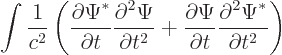or tak­ing one time de­riv­a­tive out­side the in­te­gral, that is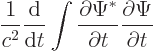That is the first needed time de­riv­a­tive, since a num­ber times its com­plex con­ju­gate is the square mag­ni­tude of that num­ber.

The sec­ond term in the Klein-Gor­don equa­tion pro­ducesThat equalsas can be seen by dif­fer­en­ti­at­ing out the par­en­thet­i­cal ex­pres­sion in the first in­te­gral with re­spect toand bring­ing the time de­riv­a­tive in the sec­ond term in­side the in­te­gral. The first in­te­gral above is zero for a pe­ri­odic box, for an im­pen­e­tra­ble con­tainer, and for in­fi­nite space for the same rea­sons as given in the de­riva­tion for the Schrö­din­ger equa­tion. The sec­ond term above is the needed sec­ond time de­riv­a­tive.

The fi­nal of the three terms in the Klein-Gor­don equa­tion pro­ducesThat equals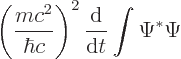as can be seen by bring­ing the time de­riv­a­tive in­side the in­te­gral. This is the last of the three needed time de­riv­a­tives.